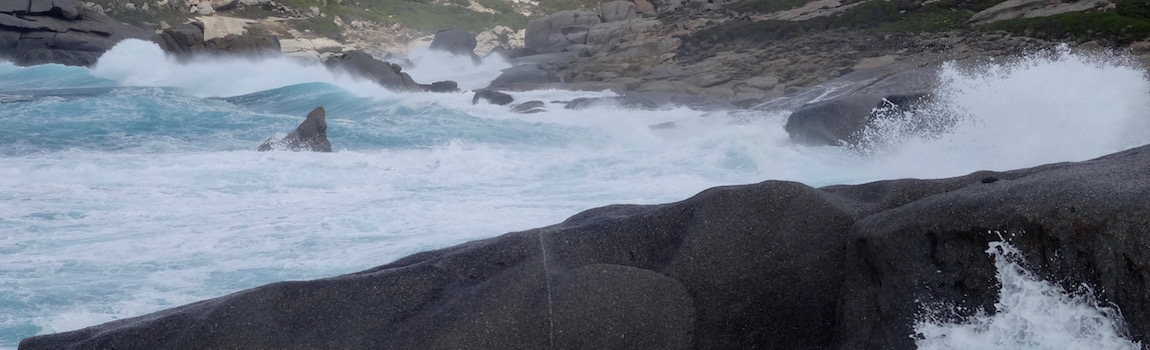Waves transfer energy from one place to another.

1 motion of waves

Row up in a line, take a rope between your hands and let the first person start to make a wave.

Make a video of this wave and try to find out most about motion of waves.

enactive

2 wave equation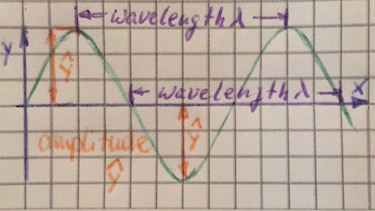Waves transfer energy from one place to another without transferring mass.

Amplitude $\bar{y}$ is the intensity of a wave. This determines the loudness of a soundwave, or the brightness of a lightwave.

The wavelength $\lambda$ is the distance bewtween the point of one wave and the exact same point of the next wave, thus for example the distance between two maximas.

The frequency $f=1/T$ of a wave is the number of waves per second.

$$y(x,t)=\hat{y} \cdot \sin\left(2\pi\left(\frac{t}{T}-\frac{x}{\lambda}\right)\right)$$
3 Transverse and longitudinal wavesSome waves must travel through a medium: sound or water. Some do not need a medium and can travel through vaccum: light, electro-magnetic, x-ray.

In transverse waves parcticles move perpendicular to the direction of the wave. In longitudinal waves particles move for- and backward in the same direction as the wave.

All electro-magnetic waves like light, sismic waves and water waves are transverse waves. Sound waves are longitudinal waves.

Polarisation occurs in transverse waves, like light.

4 speed of a wave

The speed of a wave $c$ in (m/s) is the frequency in (Hz) times the wavelength in (m).

$$c=f\cdot \lambda$$

Freqeuncy and Wave­length Ranges

 Human Hea­ring 20 Hz - 20 kHz 17 mm - 17 m Radio waves 100 MHz 3 m Wi-fi 2.4 GHz 125 mm Visible light 476 THz - 750 THz 400 nm - 630 nm
5 Hugens' Principle (1629-1695)Every point of a wavefront may be considered as a source of a secondary wavefront.

If an aperture in an obstacle is smaller then the wavelength, diffraction occurs.

sources:
K. Johnson et. al., "Advanced Physics for you", Oxford, 2015
J. Blum, Christliche Deutsche Schule Chiang Mai
Wikipedia, "Electromagnetic Spectrum"

#### Question 1 Waves and medium

• Which waves need a medium to travel, which not?
• What is the wavelength of a wave?
• What is the speed of a light wave?
• Explain the difference between wavelenght and frequency of a wave.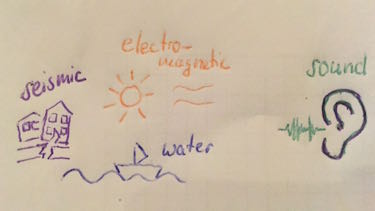• Waves which need a medium to travel: sound, water, seismic
Waves which need no medium to travel: electromagnetic waves
• The wavelength of a wave: The distance between to adjacent identical points in a wave.
• speed of a light wave: 300,000,000 m/s
• difference between wavelength and frequency: The frequency is the number of wave cycles per second.

#### Question 2 Speed of wave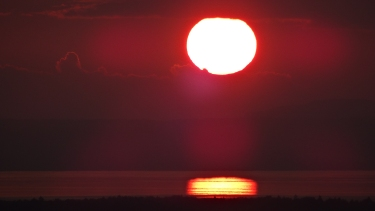Calculate the speed of different waves.

1. sound waves
2. Wi-fi waves
3. light waves
4. water waves
5. seismic waves

1. sound waves: in air: $c=340\: m/s$, in water: $c=1500\: m/s$
2. Wi-fi waves: $c=300\cdot 10^6\: m/s$
3. light waves: $c=300\cdot 10^6\: m/s$
4. water waves: depends on depth of water and the wavelength: for example with $\lambda=340\: m$ we can have $c=23\: m/s$.
5. seismic waves: $c=2000-8000\: m/s$

### First relax ...

Sound waves

"Sound waves are all around us, and when harnessed, can do some super cool things. Trace looks at a few ways we're using the power of sound waves to our advantage."

#### Question 3 time-displacement-diagram

The time-displacement-diagram shows the sound waves produced by two loudspeakers A and B.

1. Calculate the frequency f of the waves.
2. Determine the phase shift between the two waves.
3. State the type of wave produced by the speakers.
4. Reason which loudspeaker produces a louder sound.
5. Calculate the speed of the sound waves.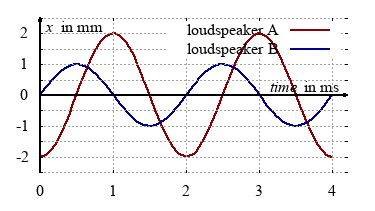1. frequency f of the waves: $f=\frac{1}{T}=\frac{1}{2\:ms}=500\:Hz$
2. phase shift between the two waves: $\varphi=90\: °$
3. Sound waves are longitudinal waves
4. The displacement or amplitude of loudspeaker A is larger. Therefore speaker A produces a louder sound.
5. wavelength of the sound waves: $\lambda=\frac{c}{f}=\frac{340\:m/s}{500\:Hz}=68\: cm$

#### Question 4 Tsunami

Tsunamis do threaten many costal regions.275,000 people lost their lives by the Tsunami occured 2004 in the Indian ocean.

1. Find out the speed and wavelength of this Tsunami wave.
2. What was the reason for the huge Tsunami?
3. Find out the vertical displacement of a Tsunami wave.
4. State why the height in shallow water increases dramatically.
1. speed: $c=200\:ms^{-1}$, wavelength: $\lambda=100\:km$
2. At the coast of Sumatra the see bed moved 15 m vertically over a length of 1500 km.
3. amplitude: $\hat{y}=1\: m$
4. In shallow water the wave slows down, so the tail of the wave catches up the front and mounts up to increased height.

### Terms and phrases

 wavelength $\lambda$ distance between two maxima of a wave frequency $f$ number of oscillations per second speed of light $c$ rate at which light travels $c=300\cdot 10^6$ m/s
 amplitude intensity of a wave displace­ment an object's change in position